# Single Dimensional Array: Declaration, Initialization and Accessing Elements

## ICSE Computer Applications

Now that we have a general idea about arrays, we will start understanding arrays from programming perspective. In this lesson we will learn about how to declare and initialize a single dimensional array and access each of its elements.

You can think of a single dimensional array as a row of numbered boxes.Each box can hold one item in it. Looking at it this way, we can say that an array is a group or a collection of same type of variables. You can create an array of any of the data types that Java provides including both primitive and reference types.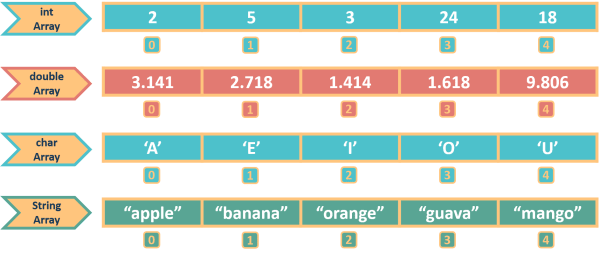## Arrays are Homogeneous

By rule, Java requires that all items in an array must be of the same type. An int array will have all int values in it, a char array will have all char values in it and so on. We cannot have an array in which the first box contains an int value, the second box contains a char value, third box contains a double value, fourth box contains a String value and so on. You will get a compilation error for this. Java does not allow this. It will not work.

## Declaring and Initializing Single Dimensional Arrays

We know how to declare and initialize variables.

`int a = 5;`

This statement declares an int variable `a` and assigns 5 to it.

`int arr[] = {1, 2, 3, 4, 5, 6};`

This is how, we declare an int array `arr` and initialize it with values from 1 to 6. In both cases we start with the data type, then we write an identifier. We named our variable `a` and our array `arr`. Like variable, name of array is also an identifier and all the identifier naming rules which we learned in Code Blocks and Tokens lesson apply to it. Then we have a pair of square brackets extra in case of arrays. It signifies that we are declaring an array variable, not a regular variable. We can place these square brackets at the end of array name as shown in the statement above or we can also place them between the data type and array name like this:

`int[] arr = {1, 2, 3, 4, 5, 6};`

Both these statements are equivalent, you can use whichever way you like.

Moving on, in the variable case we assign a single value to `a` as variables can hold only a single value. But to `arr`, we assign an array which is a group of values. All the values in the array are separated by commas and the entire array is enclosed within curly brackets.

Now that we have seen this example, we can easily write the general syntax of declaring and initializing an array like this:

```<type> <array-variable>[] = {<value1>, <value2>, ….};
OR
<type>[] <array-variable> = {<value1>, <value2>, ….};```

Below are a few examples of declaring and initializing arrays of different types:

```int arr[] = {1, 2, 3, 4, 5, 6};

double consts[] = {3.14159, 2.71828, 1.41421};

char vowels[] = {'A', 'E', 'I', 'O', 'U'};

String fruits[] = {"apple", "mango", "guava"};```

The first example shows an int array containing 6 elements. The next example is that of a double array containing 3 elements. After that we declare and initialize a char array named vowels containing the 5 vowels. The last example shows a String array containing 3 strings.

The number of elements present in the array is called the size or length of the array. Size of arr is 6 as there are 6 elements present in it. Size of consts is 3, vowels is 5 and fruits is 3.

## Accessing Array Elements

Remember, I told you that you can think of an array as a row of numbered boxes. So, you can visualize the array `arr` like this: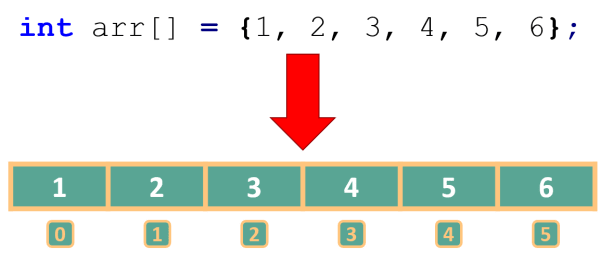To access an individual item from this array, write out the name of the array and the number of the box you want to access, inside square brackets — `arr`. It will give you the item in the array arr sitting in box number 3 which is the value 4.

The term we use in Java to refer to these box numbers is Index or Subscript. So, `arr` means give me the item present at index 3 of the array `arr`.The general syntax to access an individual element of an array is:

`<array-variable>[<index>];`

For example,

int[] arr = {1, 2, 3, 4, 5, 6};
arr ⇒ 1      arr ⇒ 4
arr ⇒ 2      arr ⇒ 5
arr ⇒ 3      arr ⇒ 6

Notice that the first index in the array is 0. In programming, we start counting from 0 not 1. It means that value at index 0 is the first item of the array, value at index 1 is the second item of the array, value at index 2 is the third item of the array and so on. Index of last element of the array is length of the array minus one. So, the index of the array ranges from 0 to array-length minus 1. We will use this a lot while accessing array elements within a loop.

The fact that array index starts at 0 and not 1 is very important. Forgetting this is the most common mistake students make in arrays. In maths, you usually start counting from 1 so you are used to that. For example, to access the 3rd element of the array `arr` shown above, you may write `arr`. But it is incorrect. `arr` will give you the fourth element of the array and not third as array index starts at 0. Third element is at index 2, fourth is at index 3 so `arr` gives fourth element. Writing `arr` when you want to access the third element of the array is incorrect. Don’t make this mistake otherwise you will lose marks. Third element is at index 2, so the correct way to access it is by writing `arr`. I will repeat this point many times in the course to make you remember. From your side, you should also make a note of this.

## Subscript, Subscripted Variable, Subscripted Value

We learnt that we can access an individual element of an array using this syntax:

`<array-name>[<index>];`

For the array `int arr[] = {1, 2, 3, 4, 5, 6};`, `arr` will give the second element of the array which is 2.Here, the 1 inside the square brackets is the called the subscript, arr is called subscripted variable and 2 which is the value present at the specified subscript is called the subscripted value.

So, we can define these terms as follows:

• Subscript — The index of the element in the array.
• Subscripted Variable — Name of the array when it is used with a subscript to access a single element of the array.
• Subscripted Value — The value of the element at that index.

## A Simple Array Program in Java BlueJ

The below program prints the number of days in each month of the year using arrays.

```public class KboatMonthDays
{
public static void main(String args[]) {

int monthDays[] = {31, 28, 31, 30, 31, 30, 31, 31, 30, 31, 30, 31};

System.out.println("There are " + monthDays + " days in January");
System.out.println("There are " + monthDays + " days in February");
System.out.println("There are " + monthDays + " days in March");
System.out.println("There are " + monthDays + " days in April");
System.out.println("There are " + monthDays + " days in May");
System.out.println("There are " + monthDays + " days in June");
System.out.println("There are " + monthDays + " days in July");
System.out.println("There are " + monthDays + " days in August");
System.out.println("There are " + monthDays + " days in September");
System.out.println("There are " + monthDays + " days in October");
System.out.println("There are " + monthDays + " days in November");
System.out.println("There are " + monthDays + " days in December");
}
}```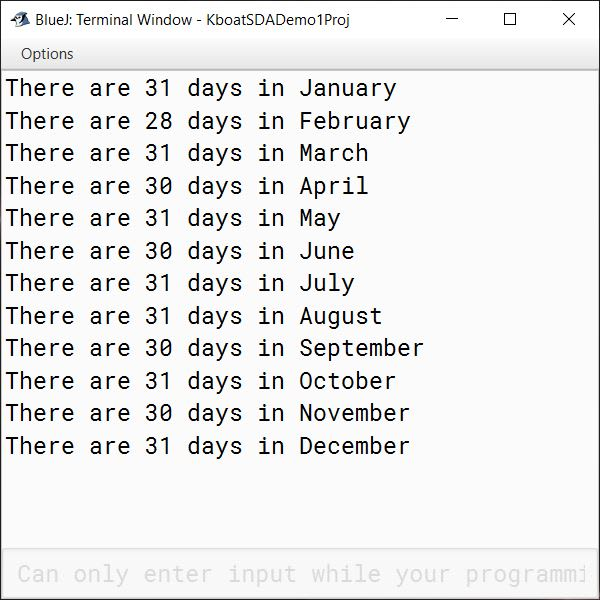In the first line of the main method:

`int monthDays[] = {31, 28, 31, 30, 31, 30, 31, 31, 30, 31, 30, 31};`

we declare an int array monthDays. Each element of this array is the number of days present in the corresponding month. You can visualize this array like this: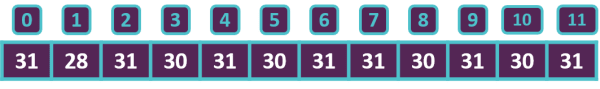This first element 31 is the number of days in January, second is the number of days in February and so on.

Next, we access each element of the array using its index to print the number of days in the corresponding month. So, `monthDays` gives us the first element 31, `monthDays` gives us the second element 28, `monthDays` gives us the third element 31 and so on. Again, I want to remind you here that index of an array begins from 0 and goes on till length of array – 1. Here it ranges from 0 to 11 as length of array `monthDays` is 12.

### Length of the Array

You can get the length of the array in your program like this — `monthDays.length`
Remember, length of the array and size of the array means the same thing. I will use length and size interchangeably.

In the below program, I have commented out the statements to print the elements of the array and added a statement to print the length of the array.

```public class KboatMonthDays
{
public static void main(String args[]) {

int monthDays[] = {31, 28, 31, 30, 31, 30, 31, 31, 30, 31, 30, 31};

System.out.println("Length/Size of monthDays array = " + monthDays.length);

// System.out.println("There are " + monthDays + " days in January");
// System.out.println("There are " + monthDays + " days in February");
// System.out.println("There are " + monthDays + " days in March");
// System.out.println("There are " + monthDays + " days in April");
// System.out.println("There are " + monthDays + " days in May");
// System.out.println("There are " + monthDays + " days in June");
// System.out.println("There are " + monthDays + " days in July");
// System.out.println("There are " + monthDays + " days in August");
// System.out.println("There are " + monthDays + " days in September");
// System.out.println("There are " + monthDays + " days in October");
// System.out.println("There are " + monthDays + " days in November");
// System.out.println("There are " + monthDays + " days in December");
}
}```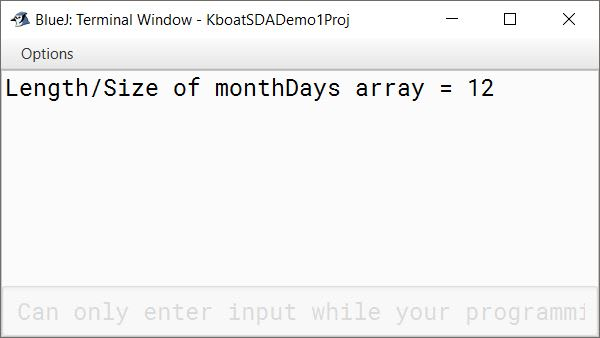In the output above, we get the length of this array as 12 as there are 12 elements in the array.

### Accessing Array Elements with Loop

Next, let’s see how we can access the elements of the array using a loop. I don’t like the 12 println statements for printing the days of the month as writing 12 of them is a lot of work. I want to do it in a shorter and simpler way. Notice the indexes of the array are going from 0 to 11. If I run a for loop from 0 to 11 then I can use the loop control variable of this for loop as the index of the array. This way I will be able to print all the elements of the array using a single print statement. Below program shows how to do this:

```public class KboatMonthDays
{
public static void main(String args[]) {

int monthDays[] = {31, 28, 31, 30, 31, 30, 31, 31, 30, 31, 30, 31};

System.out.println("Length/Size of monthDays array = " + monthDays.length);

/*
* We replaced the 12 println statements
* with a single for loop to print the
* number of days in each month
*/
for (int i = 0; i < monthDays.length; i++) {
System.out.println(monthDays[i]);
}
}
}```

Let's take a closer look at the loop we introduced:

```for (int i = 0; i < monthDays.length; i++) {
System.out.println(monthDays[i]);
}```

The loop control variable `i` is initialized to 0 as the array index starts at 0. It should go till 11 i.e. one less than the length of the array. So, we have put the condition as `i < monthDays.length`. `monthDays.length` gives us 12, `i` should be less than 12 so `i` will increment till 11 and after that the loop will stop. `i` will be incremented by 1 in each iteration. Inside the loop, we are printing the elements of the array `monthDays`.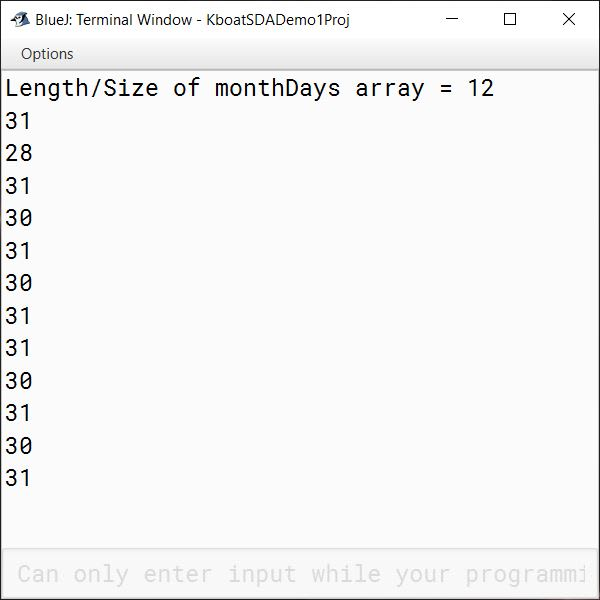The output of the program above confirms that we have successfully printed all the elements of the array using a for loop. But this output is not as pretty and verbose as the previous one. I want to get the same output as before but with this simplicity without having to use 12 println statements. How can we do that?

### Adding Another Array for Month Names

If I create another array to hold the names of the month as shown below, then I should be able to do it.

```String monthNames[] = {"January",
"February",
"March",
"April",
"May",
"June",
"July",
"August",
"September",
"October",
"November",
"December"};```

Now if I change the println statement to take the name of the month from this array `monthNames` as shown below then I should get the verbose output as before.

```for (int i = 0; i < monthDays.length; i++) {
System.out.println("There are " + monthDays[i] + " days in " + monthNames[i]);
}```

Below is the complete updated program along with the output:

```public class KboatMonthDays
{
public static void main(String args[]) {

int monthDays[] = {31, 28, 31, 30, 31, 30, 31, 31, 30, 31, 30, 31};

String monthNames[] = {"January",
"February",
"March",
"April",
"May",
"June",
"July",
"August",
"September",
"October",
"November",
"December"};

System.out.println("Length/Size of monthDays array = " + monthDays.length);

for (int i = 0; i < monthDays.length; i++) {
System.out.println("There are " + monthDays[i] + " days in " + monthNames[i]);
}
}
}```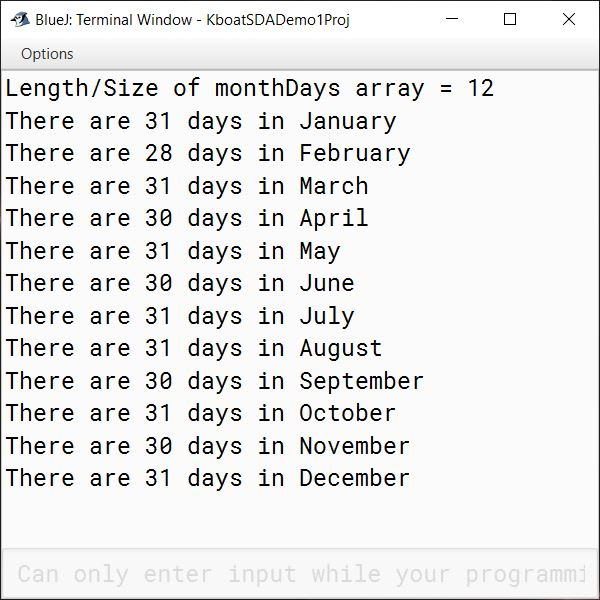### Invalid Array Index Error — ArrayIndexOutOfBoundsException

The indexes of the arrays `monthDays` and `monthNames` range from 0 to 11. What will happen if I try to access an out of range index like 12 or 13 or -1 or -5?

`System.out.println(monthDays);`
to the program. I am trying to access the element at index 12 but `monthDays` array has indexes from 0 to 11 only. The program compiles without errors and executes but when the program control comes to this newly added line, we get a runtime error.
`ArrayIndexOutOfBoundsException`
because index 12 is out of bounds for length 12. It means that the length of the array is 12 so the valid range of its indexes is from 0 to 11 and index 12 which we have specified is outside the valid range. At runtime, Java checks if all the indexes of the array we are trying to access are within the valid range of the array or not. If it finds any violations, it throws this `ArrayIndexOutOfBoundsException`. Accessing an invalid index of the array will cause a runtime error.